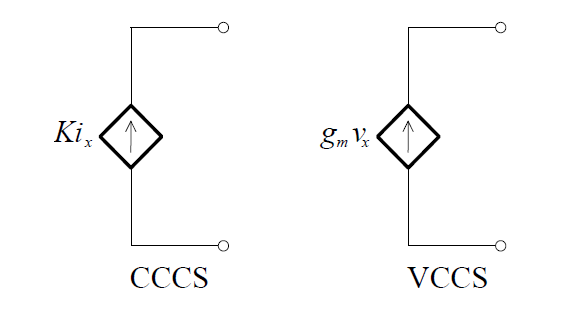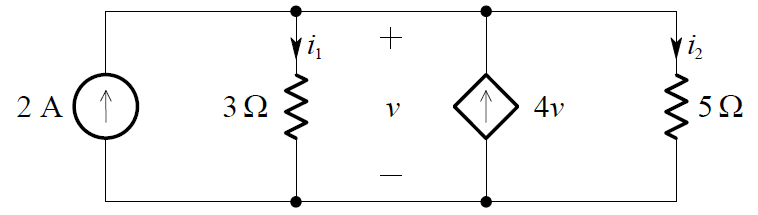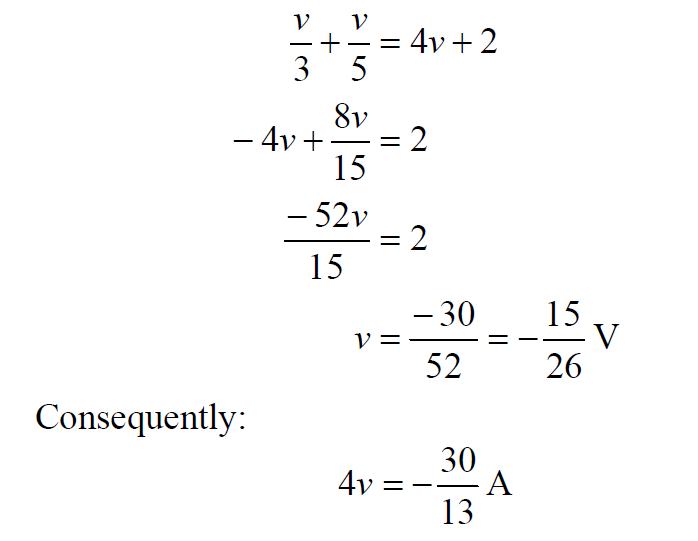# Dependent Current Source

A dependent current source establishes a current, which is independent of the voltage across it, that is determined by the voltage or current at some other location in the electrical system. There are two types of dependent current source  –  the  voltage controlled  current  source  (VCCS)  and  the  current controlled current source (CCCS).These  sources  are  mathematical  models  that  are  useful  in  modelling  real circuits and systems, e.g. they are used in modelling transistors.

Example:

Consider the circuit shown below. In this circuit the value of the dependent current source is specified by a voltage – it is a VCCS.To solve for v, we apply KCL and obtain:

i1 + i2  = 4v + 2

Thus:and this is the value of the dependent current source, in amperes. The other variables in the circuit are:Don't Miss Our Updates
Be the first to get exclusive content straight to your email.
We promise not to spam you. You can unsubscribe at any time.